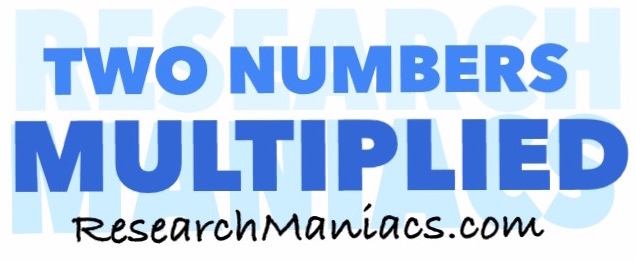What two numbers multiply to 11?What 2 numbers do you multiply to get 11? In other words, what number can you multiply with another number to get 11?

The formula to solve this would be:

X x Y = 11

There are at least two combinations of two numbers that you can multiply together to get 11.

For your convenience, we have made a list of all the combinations of two numbers multiplied by each other that will make 11:

1 x 11 = 11
11 x 1 = 11

Two Numbers Multiplied
Enter another number below to see which combinations of two numbers multiplied will equal that number.

What two numbers multiply to 12
Go here for the next number on our list.